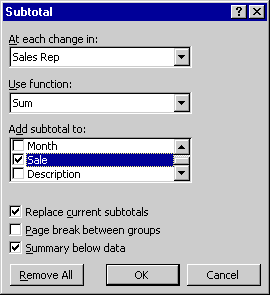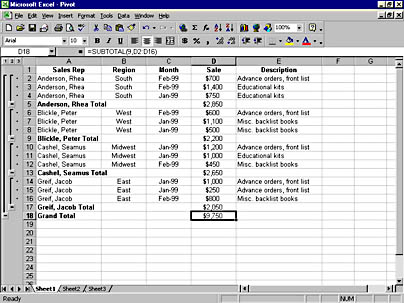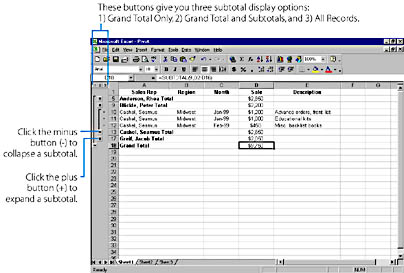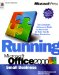# Using the Subtotals Command to Organize a List

The Subtotals command on the Data menu helps you to organize a list by displaying records in groups and adding subtotals, averages, or other summary information. The Subtotals command can also display a grand total at the top or bottom of your list, letting you quickly add up columns of numbers. As a bonus, Subtotals displays your list in Outline view so that you can expand or shrink each section in the list simply by clicking.

1. Arrange the list so that the records for each group are located together. An easy way to do this is to sort on the field on which you're basing your groups. For example, you could sort based on employee, region, or store.
2. From the Data menu, choose Subtotals. Excel opens the Subtotal dialog box and selects the list.
3. In the At Each Change In list box, choose a group whose subtotal you want to define. This should be the same column that you sorted the list with. Each time this value changes, Excel will insert a row and compute a subtotal for the numeric fields in this group of records.
4. In the Use Function list box, choose a function to use in the subtotal. SUM is the most popular, but other options are available, as described in Table 22-1.
5. In the Add Subtotal To list box, choose the column or columns to use in the subtotal calculation. You can subtotal more than one column by selecting multiple boxes, but be sure to remove any check marks that you don't want. The following screen shows the settings for a typical use of the Subtotals command:
6.7. Click OK to add the subtotals to the list. You'll see the following screen, complete with subtotals, outlining, and a grand total.
8.Table 22-1. Summary Functions in the Subtotal Dialog Box

Function Description
SUM Add up the numbers in the subtotal group.
COUNT Count the number of nonblank cells in the group.
AVERAGE Calculate the average of the numbers in the group.
MAX Display the largest number in the group.
MIN Display the smallest number in the group.
PRODUCT Multiply together all the numbers in the group.
COUNT NUMS Count the number of cells containing numeric values in the group.
STDDEV Estimate the standard deviation based on a sample.
STDDEVP Calculate the standard deviation for an entire population.
VAR Estimate the variance in the group based on a sample.
VARP Calculate the variance for an entire population.

TIP
You can choose the Subtotals command as often as necessary to modify your groupings or calculations. When you have finished using the Subtotals command, click Remove All in the Subtotal dialog box.

## Working in Outline View

When you use the Subtotals command in Excel to create outlines, you can examine different parts of a list by clicking buttons in the left margin, as shown in Figure 22-7. (Note that this is similar to the way Outline view functions in Microsoft Word.)

Click the numbers at the top of the left margin to choose how many levels of data you want to see. Click the plus or minus button to expand or collapse specific subgroups of data.Figure 22-7. The Subtotals command creates an outline view of your list.Running Microsoft Office 2000
ISBN: 1572319585
EAN: 2147483647
Year: 2005
Pages: 228